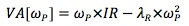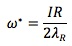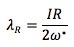### Seeing is believing!

Before you order, simply sign up for a free user account and in seconds you'll be experiencing the best in CFA exam preparation.

### Subject 4. The optimal level of residual risk

Given the opportunities described by the information ratio, the active manager should choose the portfolio that maximizes value added.

The value added curve is a curve that is tangent to the residual frontier at portfolio P* (i.e. the optimal portfolio).

Mathematically:As the risk increases, both the expected return and the penalty for risk increase.

The optimal level of residual risk (ω*) which maximizes VA[ωP] isThe optimal level of residual risk increases if:

• There are more opportunities.
• There is less residual risk aversion.

The implied level of residual risk aversion:#### Practice Question 1

Which factors determine the manager's optimal level of residual risk?

I. aggressiveness.
II. information ratio.
III. residual risk aversion.
IV. loss in alpha.

#### Practice Question 2

If the information ratio is 1 and residual risk aversion is 0.05, the optimal level of residual risk is:

A. 5%.
B. 10%.
C. 20%.

IR/(2λ) = 1/(2x0.05) = 10.

#### Practice Question 3

__________, the higher the level of aggressiveness.

I. The greater our opportunities
II. The less our opportunities
III. The higher the residual risk aversion
IV. The lower the residual risk aversion

#### Practice Question 4

A risk aversion (λ) of 0.05 usually indicates a ______ residual risk aversion.

A. restrained.
B. moderate.
C. aggressive.

#### Practice Question 5

If the information ratio is 0.75 and residual risk aversion is 0.10, the optimal level of residual risk is:

A. 3.75%.
B. 3.33%.
C. 7.50%.